Ex 4.3

Chapter 4 Class 10 Quadratic Equations (Term 2)
Serial order wise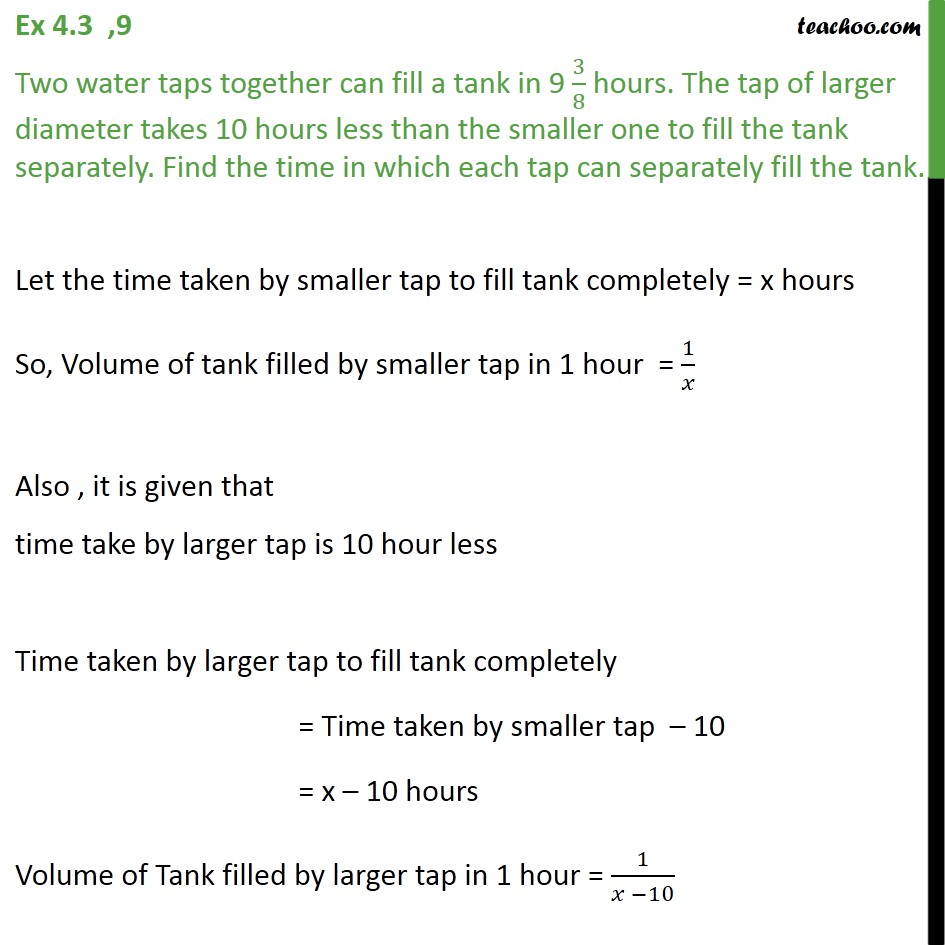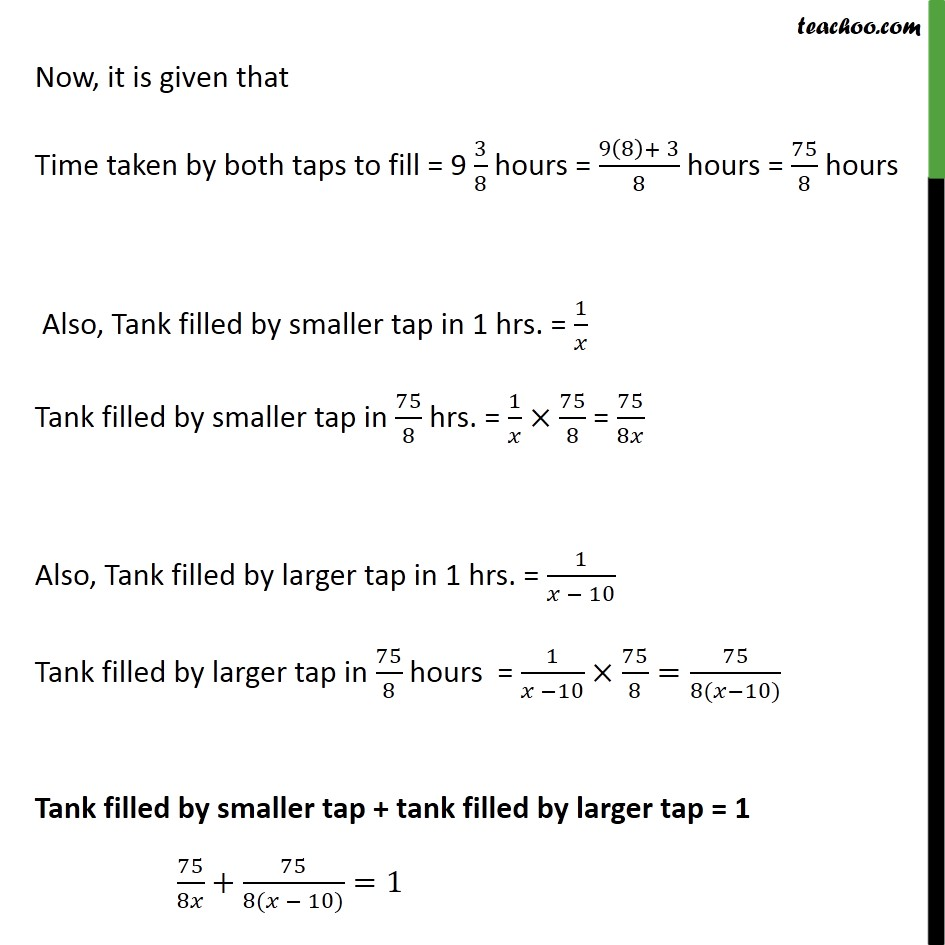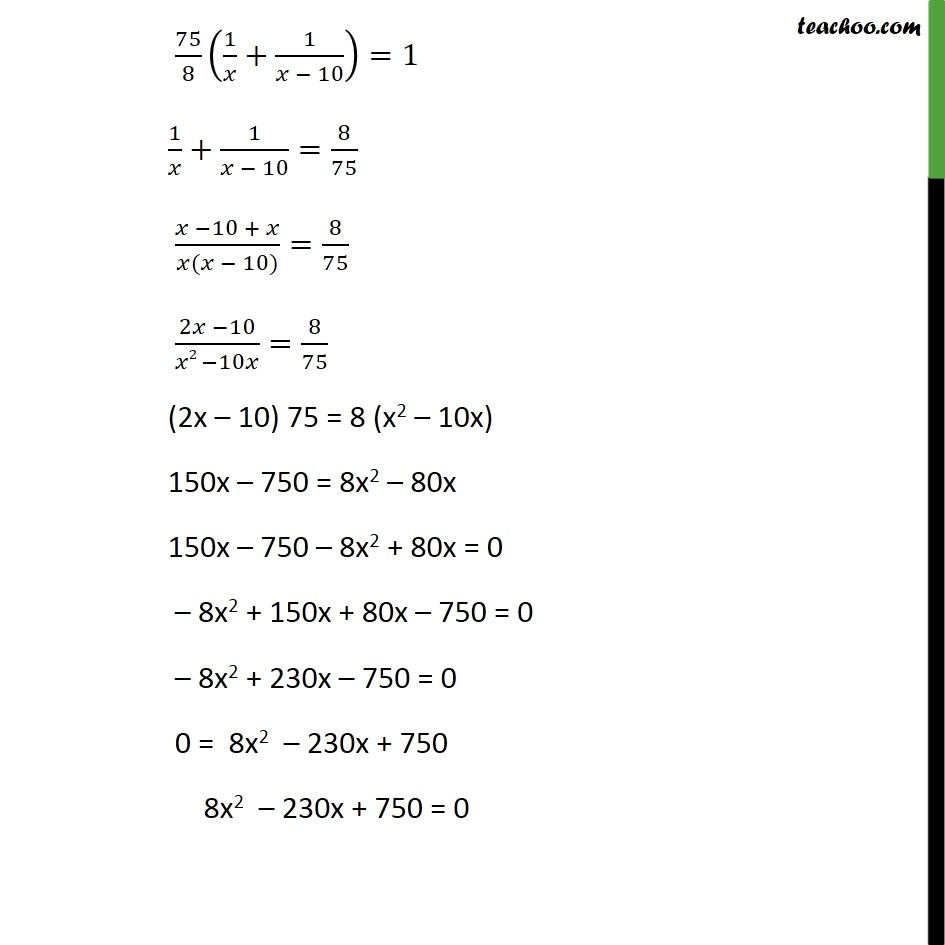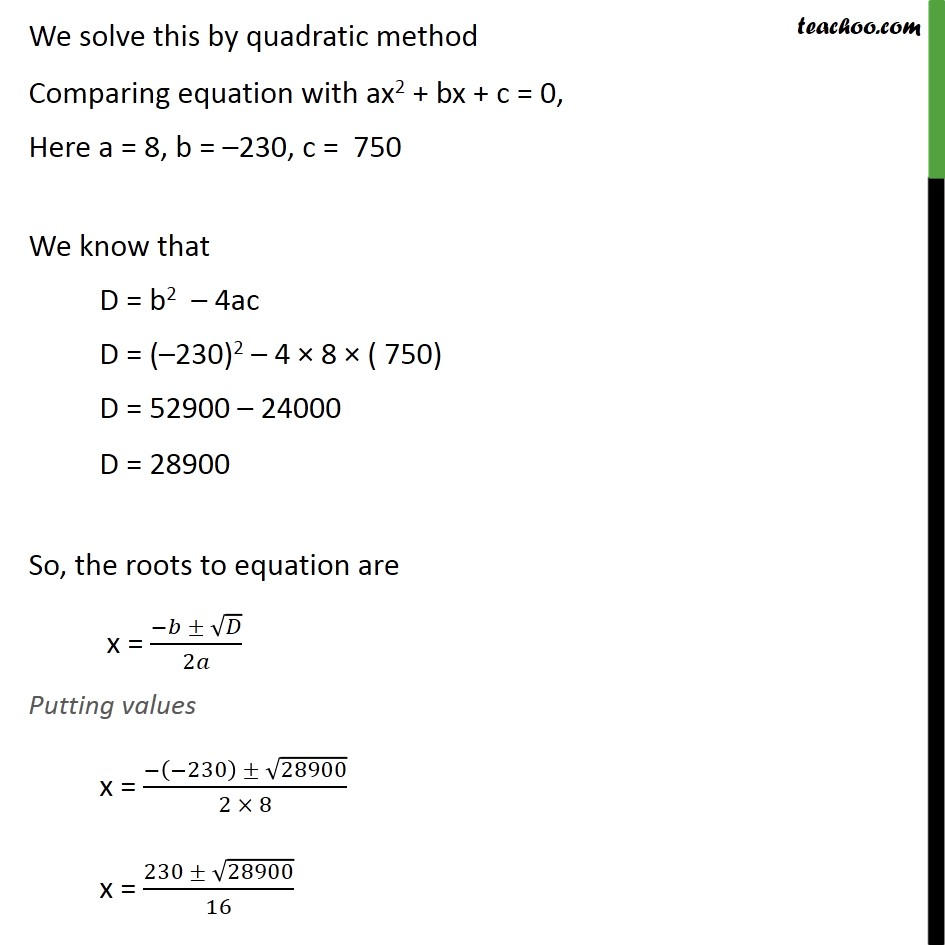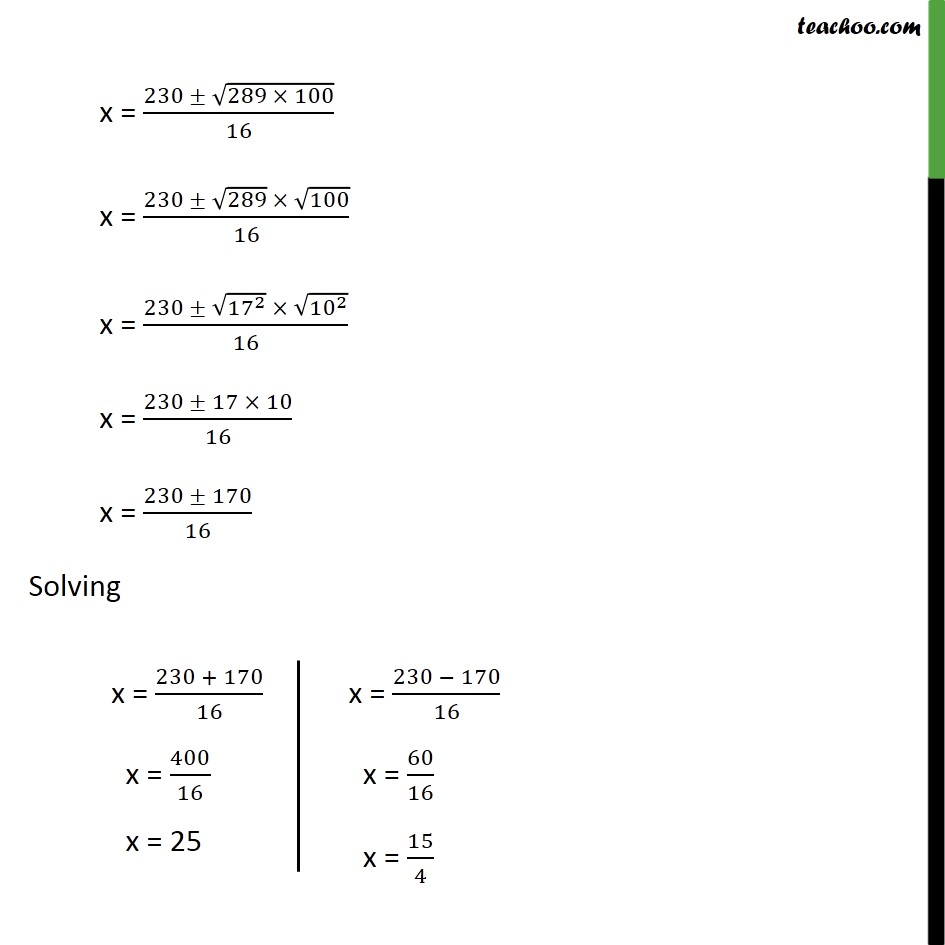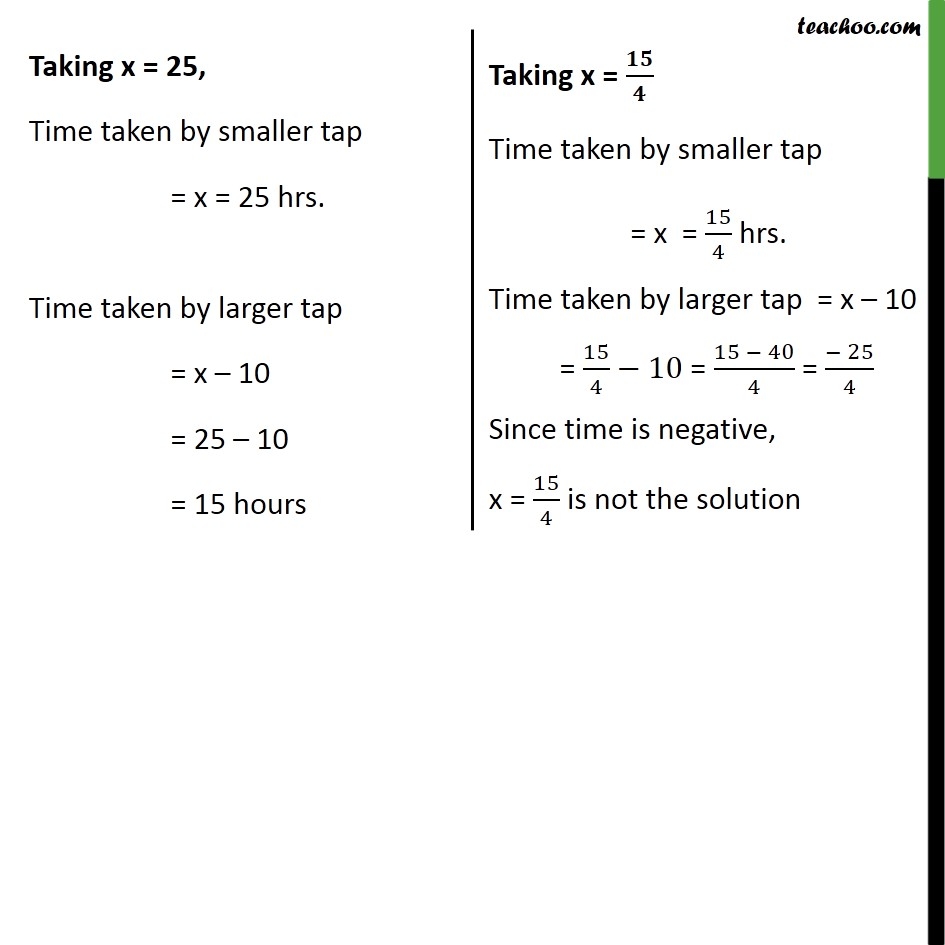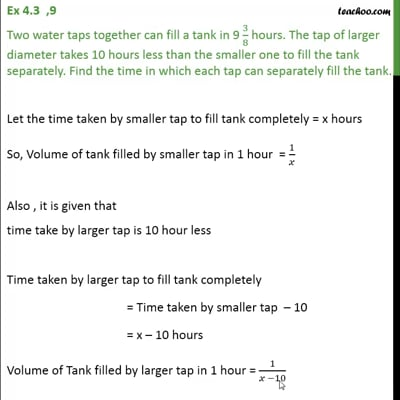This video is only available for Teachoo black users

### Transcript

Ex 4.3 ,9 Two water taps together can fill a tank in 9 3/8 hours. The tap of larger diameter takes 10 hours less than the smaller one to fill the tank separately. Find the time in which each tap can separately fill the tank. Let the time taken by smaller tap to fill tank completely = x hours So, Volume of tank filled by smaller tap in 1 hour = 1/𝑥 Also , it is given that time take by larger tap is 10 hour less Time taken by larger tap to fill tank completely = Time taken by smaller tap – 10 = x – 10 hours Volume of Tank filled by larger tap in 1 hour = 1/(𝑥 −10) Now, it is given that Time taken by both taps to fill = 9 3/8 hours = (9(8)+ 3)/8 hours = 75/8 hours Also, Tank filled by smaller tap in 1 hrs. = 1/𝑥 Tank filled by smaller tap in 75/8 hrs. = 1/𝑥×75/8 = 75/8𝑥 Also, Tank filled by larger tap in 1 hrs. = 1/(𝑥 − 10) Tank filled by larger tap in 75/8 hours = 1/(𝑥 −10)×75/8=75/(8(𝑥−10)) Tank filled by smaller tap + tank filled by larger tap = 1 75/8𝑥+75/(8(𝑥 − 10))=1 75/8 (1/𝑥+1/(𝑥 − 10))=1 1/𝑥+1/(𝑥 − 10)=8/75 (𝑥 −10 + 𝑥)/(𝑥(𝑥 − 10))=8/75 (2𝑥 −10)/(𝑥2 −10𝑥)=8/75 (2x – 10) 75 = 8 (x2 – 10x) 150x – 750 = 8x2 – 80x 150x – 750 – 8x2 + 80x = 0 – 8x2 + 150x + 80x – 750 = 0 – 8x2 + 230x – 750 = 0 0 = 8x2 – 230x + 750 8x2 – 230x + 750 = 0 We solve this by quadratic method Comparing equation with ax2 + bx + c = 0, Here a = 8, b = –230, c = 750 We know that D = b2 – 4ac D = (–230)2 – 4 × 8 × ( 750) D = 52900 – 24000 D = 28900 So, the roots to equation are x = (−𝑏 ± √𝐷)/2𝑎 Putting values x = (−(−230) ± √28900)/(2 × 8) x = (230 ± √28900)/16 x = (230 ± √(289 × 100))/16 x = (230 ± √289 × √100)/16 x = (230 ± √(〖17〗^2 ) × √(〖10〗^2 ))/16 x = (230 ± 17 × 10)/16 x = (230 ± 170)/16 Solving